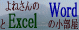# セルの値に応じてセルに色を付ける:Excel VBA入門

 スポンサードリンク

## はじめに

• セルの値に応じて色をつけたい(セルを塗りつぶしたい)ときは条件付き書式が利用できます。
ただし、Excel2003までの条件付き書式では3条件までしか設定ができませんので、4条件など条件が多いときにはVBAを利用します。
• ここではVBAを使ってセルを塗りつぶす例(条件数5個)を書いています。

## セルの値が一箇所だけ変わる場合

• E列の値が1〜5の場合、A列〜E列のセルをそれぞれの色で塗りつぶします。
• コードはワークシートのモジュールに書きます。
• シートタブでシート見出しを右クリックして「コードの表示」を実行するとVBEが開きます。
下図ではSheet1にコードが書いてある例です。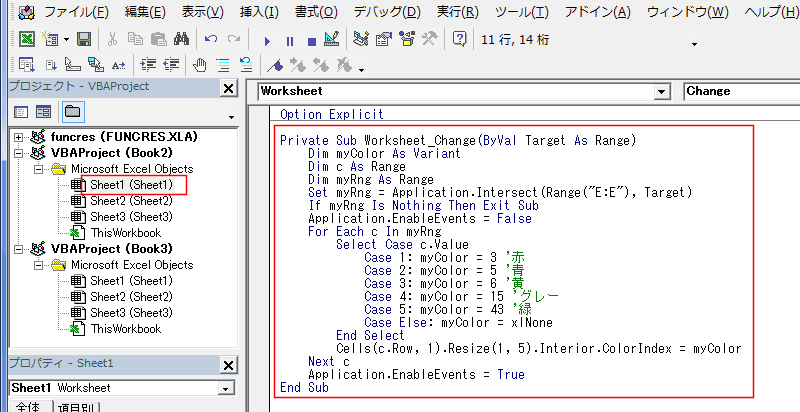• 【コード例】
• セルの色は　.Interior.ColorIndex とカラーインデックスで指定しています。
インデックス番号と色の関係は　ColorIndexと色の見本　をご覧ください。
•  Private Sub Worksheet_Change(ByVal Target As Range) 　Dim myColor As Variant 　　If Target.Count <> 1 Then Exit Sub 　　If Target.Column <> 5 Then Exit Sub 　　Application.EnableEvents = False 　　Select Case Target.Value 　　　　Case 1 　　　　　　myColor = 3 '赤 　　　　Case 2 　　　　　　myColor = 5 '青 　　　　Case 3 　　　　　　myColorl = 6 '黄 　　　　Case 4 　　　　　　myColor = 15 'グレー 　　　　Case 5 　　　　　　myColor = 43 '緑 　　　　Case Else 　　　　　　myColor = xlNone 　　End Select 　　Cells(Target.Row, 1).Resize(1, 5).Interior.ColorIndex = myColor 　　Application.EnableEvents = True End Sub
• 実行後の例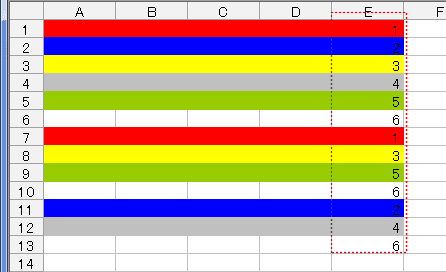## セルの値が複数箇所変わる場合

• 変化したセルがE列と重なっているか否かを判断して、E列の値が変化していたら動作します。
• 【コード例】
•  Private Sub Worksheet_Change(ByVal Target As Range) 　Dim myColor As Variant 　Dim c As Range 　Dim myRng As Range 　　Set myRng = Application.Intersect(Range("E:E"), Target) 　　If myRng Is Nothing Then Exit Sub 　　Application.EnableEvents = False 　　For Each c In myRng 　　　　Select Case c.Value 　　　　　　Case 1 　　　　　　　　myColor = 3 '赤 　　　　　　Case 2 　　　　　　　　myColor = 5 '青 　　　　　　Case 3 　　　　　　　　myColorl = 6 '黄 　　　　　　Case 4 　　　　　　　　myColor = 15 'グレー 　　　　　　Case 5 　　　　　　　　myColor = 43 '緑 　　　　　　Case Else 　　　　　　　　myColor = xlNone 　　　　End Select 　　　　Cells(c.Row, 1).Resize(1, 5).Interior.ColorIndex = myColor 　　Next c 　　Application.EnableEvents = True End Sub

## ColorIndexと色の見本

• ColorIndexと色の見本
• ColorIndexとRGB値の関係
ColorプロパティでR(赤),G(緑),B(青)を調べることができます。
また逆に、RGB値で色を指定することができます。
 Sub iro_Test() Dim R As Integer Dim G As Integer Dim B As Integer Dim MyColor As Long Dim i As Long 　For i = 2 To 57 　　MyColor = Cells(i, 2).Interior.Color 　　R = MyColor Mod 256 　　G = Int(MyColor / 256) Mod 256 　　B = Int(MyColor / 256 / 256) 　　Cells(i, 3).Value = "RGB(" & R & "," & G & "," & B & ")" 　Next i End Sub

見やすいように3分割して並べています。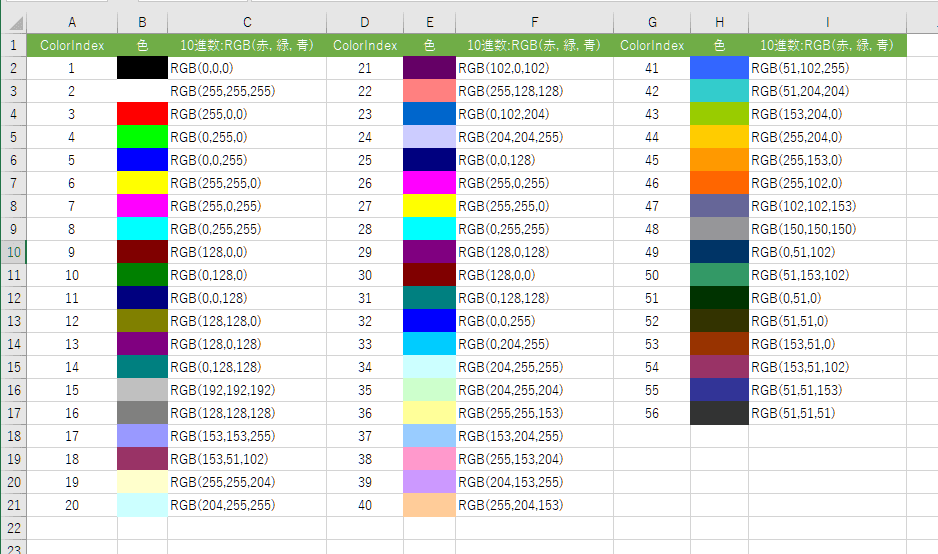• セルを選択して、[Ctrl]+ でセルの書式設定 ダイアログボックスを表示して、塗りつぶしタブを選択します。
[その他の色]ボタンをクリックして、色の設定 ダイアログボックスを開きます。
[ユーザー設定]タブを選択すると、RGBの各値を調べることができます。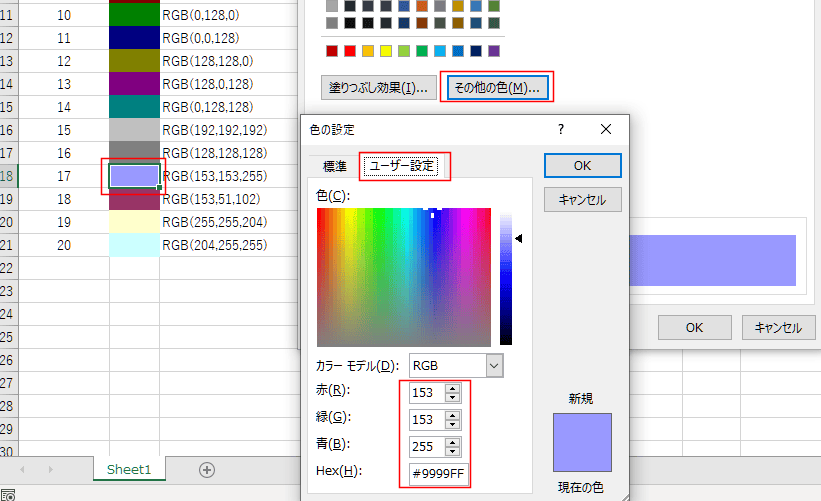スポンサードリンク

よねさんのWordとExcelの小部屋Excel(エクセル) VBA入門：目次｜セルの値に応じてセルに色を付ける

PageViewCounter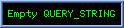Since2006/2/27